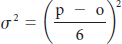# Probability of completing the project within given time

An advantage of using probabilistic time estimates is the ability to predict the probability of project completion dates. We learned how to calculate the expected time for each activity with the three time estimates provided. Now we need to calculate the variance for each activity. The variance of the beta probability distribution for each activity isWhere

p = pessimistic activity time estimate

o = optimistic activity time estimate

## 2 thoughts on “Probability of completing the project within given time”

error: Content is protected !!